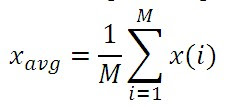# M-point moving average filter MATLAB Program

Hi friends, today we are going to learn a how to write a MATLAB program for m point moving average filter.

## MATLAB Program for M-point moving average filter

Steps for writing M-point moving average filter program in MATLAB:

1. Take a given signal 2n*(0.9)^n and denote it by s(n).

2. Then generate a random noise signal having the same length (50) as that of s(n) and denote it by d.

3. Then add s+d and save it in p, i.e. p=s+d.

4. We know that the formula for m point moving average filter is given by5. To determine summation use one for loop.

6. Finally, divide summation by M, you will get original signal.

### The MATLAB program:

`clc;clear all;close all;n=1:50;s(n)=2*n.*(0.9).^n;subplot(2,2,1)stem(s)xlabel('Original signal')d=rand(1,50);subplot (2,2,2)stem(d)xlabel('Noise signal')p=s+d;subplot (2,2,3)stem(p)xlabel('Corrupted Signal')m=100;xu=0;for i=1:md=rand(1,50);x=s+d;xu=xu+x;endxu=xu/m;subplot(2,2,4)stem(xu)xlabel('Output signal')`

OUTPUT of M-Point moving average filter :